# Graph Practice Questions – Test 1

##### 1. In the following figure, what is the area of the shaded circle inside of the square?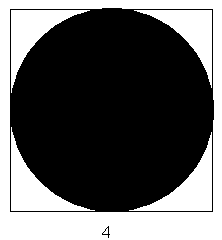1. 512
2. 256
3. 16
4. 50.24
5. 12.57
##### 2. In the figure below, determine the area of the shaded region of the figure.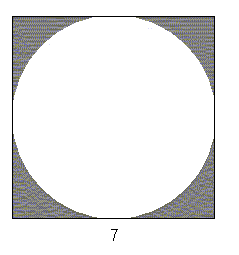1. 9.354
2. 10.52
3. 16.437
4. 49
5. 104.86

##### 3. What are the coordinates of point A on the following graph?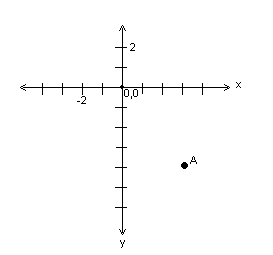1. (-3, -4)
2. (-4, 3)
3. (3, -4)
4. (-4, -3)
5. (3, 4)
##### 4. What was the average number of babies that Dr. Jones delivered each year from 1995 to 1998?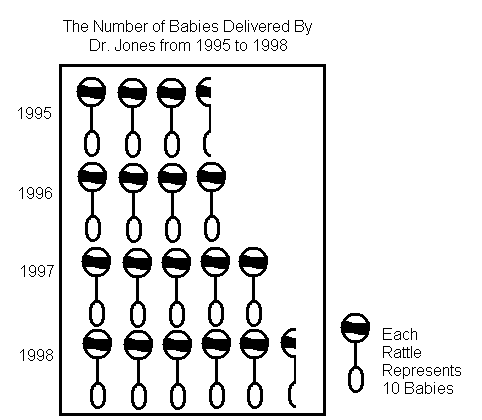1. 35
2. 40
3. 45
4. 50
5. 55
1. 25
2. 35
3. 45
4. 55
5. 65
1. 6 ½
2. 7
3. 7 ½
4. 8
5. 8 ½
##### 7. If XYZ Auto Company sold 23,000 vehicles in 1999, how many were SUV’s?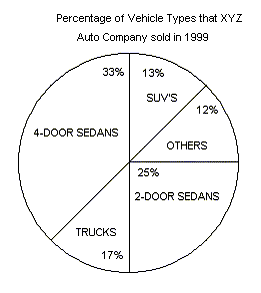1. 2,990
2. 3,030
3. 3,450
4. 4,760
5. 4,775
1. 35,000
2. 40,000
3. 45,000
4. 50,000
5. 55,000
1. 3,578
2. 4,950
3. 5,120
4. 5,670
5. 5,845
##### 10. How much did the infant gain in the first month of life?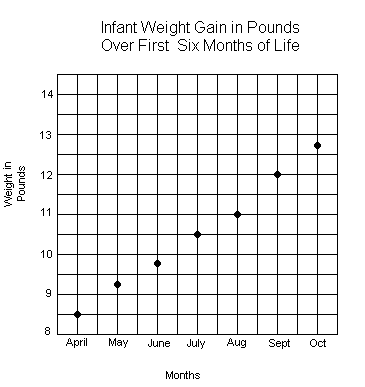1. 6 ounces
2. 12 ounces
3. 15 ounces
4. 8 lbs 8 ounces
5. 9 lbs 4 ounces
##### 11. What was the average weight of the infant from April to October, rounded to the nearest ounce?
1. 10 lbs
2. 10 lbs 5 ounces
3. 10 lbs 9 ounces
4. 11 lbs 5 ounces
5. 11 lbs 9 ounces
##### 12. Between which two months did the infant gain the most weight?
1. April and May
2. June and July
3. July and August
4. August and September
5. September and October
##### 13. In the graph below, no axes or origin is shown. If point B’s coordinates are (10,3), which of the following coordinates would most likely be A’s?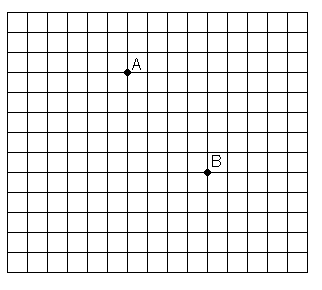1. (17, -2)
2. (10, 6)
3. (6, 8)
4. (-10, 3)
5. (-2, -17)
##### 14. How many boys attended the 1995 convention?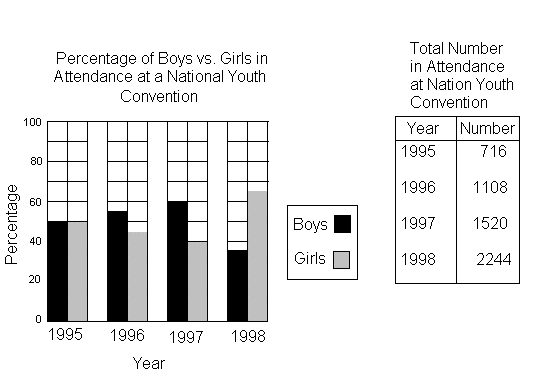1. 358
2. 390
3. 407
4. 540
5. 716
1. 1995
2. 1996
3. 1997
4. 1998
5. None
##### 16. Which two years did the least number of boys attend the convention?
1. 1995 and 1996
2. 1995 and 1998
3. 1996 and 1997
4. 1996 and 1992
5. 1997 and 1998

###### 1. E

The area of the circle may be found by using the formula, A=πr2. Since the square has a diameter of 4, the circle has a radius of 2. Substituting 2 for r, into the formula above, gives A=π(2)2, or A=4π. Thus, the area of the circle is approximately 12.57 square units.

###### 2. B

The area of the square is equal to 72, or 49, square units. The area of the circle may be represented as π(3.5)2, or 12.25π, which is approximately 38.48 square units. The area of the shaded portion of the figure equals the difference of 49 square units and 38.48 square units, or 10.52 square units.

###### 3. C

The point represents the x-value of 3 and the y-value of -4, thus the ordered pair may be written as (3, -4).

###### 4. C

The average may be written as (35+40+50+55)/4, which equals 45.

###### 5. D

Since each rattle represents the delivery of 10 babies, 5 1/2 rattles represents the delivery of 55 babies.

###### 6. E

Delivery of 85 babies would be represented by 8 whole rattles and 1/2 of another rattle, since 8 1/2 . 10=85.

###### 7. A

The number of SUVs sold is equal to 0.13 x 23,000, or 2,990.

###### 8. C

If 7,650 trucks are sold, which constitutes 17% of the total number of vehicles sold, then the total number of vehicles sold may be determined by solving the equation, 7650 = 0.17x. Dividing both sides of the equation by 0.17 gives 45,000.

###### 9. B

The total number of vehicles sold may be determined by solving the following equation for x: 0.25x = 3,750. Thus, 15,000 vehicles were sold. The number of 4-door sedans is equal to the product of 0.33 and 15,000, or 4,950.

###### 10. B

The weight increased from 8.5 pounds to 9.25 pounds, showing an increase of 0.75 pounds. The number of pounds may be converted to ounces by writing and solving the following proportion: 0.75/x=1/16. Thus, the infant gained 12 ounces during the first month of life.

###### 11. C

The average weight may be represented as the ratio, (8.5+9.25+9.75+10.5+11+12+12.75)/7, which is approximately 10.54 pounds, or 10 pounds, 9 ounces.

###### 12. D

The infant gained 1 pound between August and September, which was the greatest increase.

###### 13. C

A movement of 4 units to the left and 5 units up, from the point, (10, 3) gives (6, 8), since 10 – 4 = 6 and 3 + 5 = 8.

###### 14. A

The number of boys who attended the 1995 convention may be represented as 0.50(716), which equals 358.

###### 15. A

In 1995, 50% of the attendees were boys and 50% were girls, thus the same number of boys and girls attended the convention.

###### 16. A

The number of boys attending the convention, from 1995 to 1998, may be represented by the expressions, 0.50(716), 0.55(1108), 0.60(1520), and 0.35(2244). Thus, the years of 1995 and 1996 had the least number of boys in attendance, with numbers of 358 and 610, respectively.